# hihoCoder week 52-1-连通性·一

hihoCoder week 52-1-连通性·一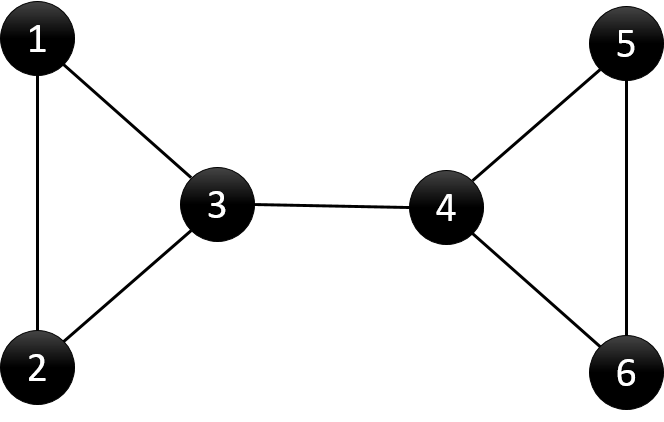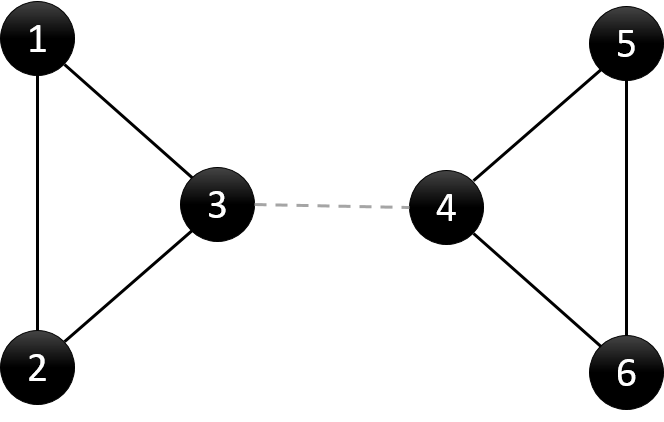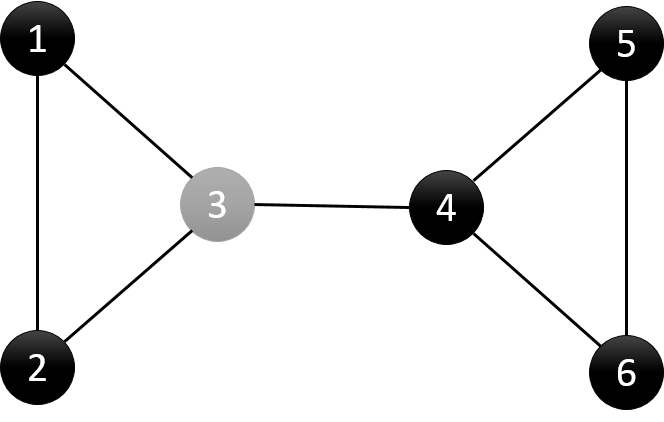6 7
1 2
1 3
2 3
3 4
4 5
4 6
5 6

3 4
3 4#include<iostream>
#include<cstdio>
#include<set>
#include<algorithm>
#include<vector>
using namespace std;
const int kMaxN = 20005;
int n, m;
typedef struct edge
{
int x, y;
bool operator<(const edge& p)const//如果要加入到set中，需要重载<
{
return (this->x<p.x) || ((this->x == p.x) && (this->y<p.y));
}
};
set<int> points;
set<edge> edges;
vector<vector<int>> graph;
int visit[kMaxN];
int dfn[kMaxN];
int low[kMaxN];
int parent[kMaxN];
void dfs(int u) {
//记录dfs遍历次序
static int counter = 0;
//记录节点u的子树数
int children = 0;
visit[u] = 1;
//初始化dfn与low
dfn[u] = low[u] = ++counter;
for (int i = 0; i < graph[u].size();i++) {
int v = graph[u][i];
//节点v未被访问，则(u,v)为树边
if (visit[v]==0) {
children++;
parent[v] = u;
dfs(v);
low[u] = min(low[u], low[v]);
//case (1)
if (parent[u] == 0 && children > 1) {
points.insert(u);
}
//case (2)
if (parent[u] != 0 && low[v] >= dfn[u]) {
points.insert(u);
}
//bridge
if (low[v] > dfn[u]) {
edge e;
e.x = u;
e.y = v;
if (u > v)
{
e.x = v;
e.y = u;
}
edges.insert(e);
}
}
//节点v已访问，则(u,v)为回边
else if (v != parent[u]) {
low[u] = min(low[u], dfn[v]);
}
}
}
int main()
{
scanf("%d %d", &n, &m);
graph.resize(n + 1);
int u, v;
while (m--)
{
scanf("%d %d", &u, &v);
graph[u].push_back(v);
graph[v].push_back(u);
}
dfs(1);
if (points.size() == 0)
printf("Null\n");
else
{
set<int>::iterator it = points.begin();
while (it != points.end())
{
printf("%d ", *it);
it++;
}
printf("\n");
}
if (edges.size() > 0)
{
set<edge>::iterator it = edges.begin();
while (it != edges.end())
{
printf("%d %d\n", (*it).x, (*it).y);
it++;
}
}
return 0;
}


# hihoCoder week 51-1-欧拉路·三

hihoCoder week 51-1-欧拉路·三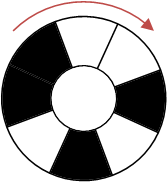——By 无名的冒险者3

00010111

#include<iostream>
#include<cstdio>
#include<cstring>
using namespace std;
const int kMaxN = 16;
int edge[1 << kMaxN];
int path[1 << kMaxN];
int path_size = 0;
int n;
void MakeGraph()
{
memset(edge, -1, sizeof(edge));
int nn = 1 << (n - 1);
for (int i = 0; i < nn; i++)
{
edge[i] = (i << 1)&(nn - 1);
edge[i] = edge[i] + 1;
}
}
void DFS(int p)
{
for (int i = 0; i < 2; i++)
{
int q = edge[p][i];
if (q != -1)
{
edge[p][i] = -1;
DFS(q);
}
}
path[path_size++] = p;
}
int main()
{
scanf("%d", &n);
if (n == 1)
{
printf("01");
return 0;
}
else if (n == 2)
{
printf("1100");
return 0;
}
MakeGraph();
DFS(0);
while (--path_size)
printf("%d", path[path_size] & 1);
return 0;
}


# hihoCoder 1121-二分图一•二分图判定

hihoCoder 1121-二分图一•二分图判定
#1121 : 二分图一•二分图判定

2
5 5
1 2
1 3
3 4
5 2
1 5
5 5
1 2
1 3
3 4
5 2
3 5

Wrong
Correct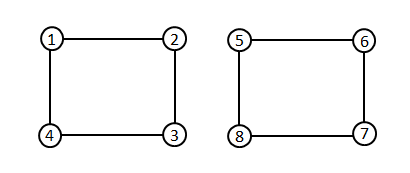#include<iostream>
#include<cstdio>
#include<vector>
#include<queue>
using namespace std;
int n, m;
vector<int> colored;
vector<vector<int>> path;
bool BFS(int start)
{
colored[start] = 0;
int num_colored = 1;
queue<int> point;
point.push(start);
while (!point.empty())
{
int current = point.front();
point.pop();
for (int i = 0; i < path[current].size(); i++)
{
if (colored[path[current][i]] == -1)
{
if (colored[current] == 0)
colored[path[current][i]] = 1;
else if (colored[current] == 1)
colored[path[current][i]] = 0;
num_colored++;
point.push(path[current][i]);
}
else if (colored[current] == colored[path[current][i]])
return false;
}
}
return true;
}
bool Check()
{
for (int i = 1; i <= n; i++)
if (colored[i] == -1)
if (!BFS(i))
return false;
return true;
}
int main()
{
int t,u,v;
scanf("%d", &t);
while (t--)
{
scanf("%d %d", &n, &m);
colored.clear();
colored.resize(n + 1, -1);
path.clear();
path.resize(n + 1);
for (int i = 0; i < m; i++)
{
scanf("%d %d", &u, &v);
path[u].push_back(v);
path[v].push_back(u);
}
if (Check())
printf("Correctn");
else
printf("Wrongn");
}
return 0;
}


# hihoCoder week 50-1-欧拉路·二

hihoCoder week 50-1-欧拉路·二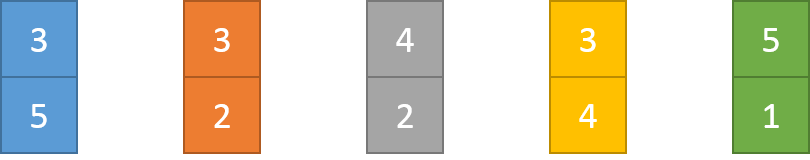——By 无名的冒险者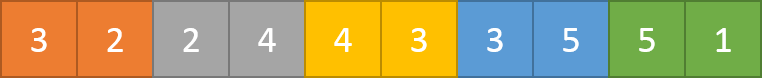5 5
3 5
3 2
4 2
3 4
5 1

1 5 3 4 2 3

DFS(u):
While (u存在未被删除的边e(u,v))
删除边e(u,v)
DFS(v)
End
PathSize ← PathSize + 1
Path[ PathSize ] ← u


#include<iostream>
#include<cstdio>
using namespace std;
const int kMaxN = 1005,kMaxM=5005;
int n, m,path_size=0;
int path[kMaxM];//是kMaxM而非kMaxN
int edge[kMaxN][kMaxN];
void dfs(int p)
{
for (int i = 1; i <= n; i++)
{
if (edge[p][i]>0)
{
edge[p][i]--;
edge[i][p]--;
dfs(i);
}
}
path[path_size++] = p;
}
int main()
{
//freopen("input.txt", "r", stdin);
scanf("%d %d", &n, &m);
int u, v;
for (int i = 0; i < m; i++)
{
scanf("%d %d", &u, &v);
edge[u][v]++;//防止有重边
edge[v][u]++;
}
dfs(1);
for (int i = 0; i < path_size; i++)
printf("%d ", path[i]);
return 0;
}


AC之后我还尝试了先找出欧拉通路的起点，再从起点开始dfs的方法，这种方法并没有更快，因为不论是从哪个点开始dfs，每个点的for循环都要完整执行，所以时间并不会减少，反而会由于寻找起点而增加时间。

# hihoCoder week 49-1-欧拉路·一

hihoCoder week 49-1-欧拉路·一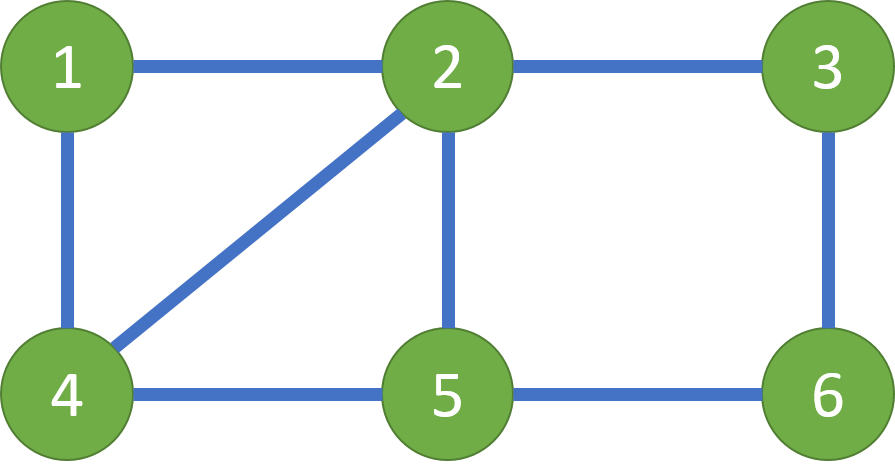6 8
1 2
1 4
2 4
2 5
2 3
3 6
4 5
5 6

Full

#include<iostream>
#include<cstdio>
using namespace std;
const int kMax = 10005;
int n, m;
int degree[kMax];
int father[kMax];
int Find(int x)
{
return x == father[x] ? x : (father[x] = Find(father[x]));
}
void Union(int x, int y)
{
father[Find(x)] = Find(y);
}
void Init()
{
for (int i = 1; i <= n; i++)
father[i] = i;
}
bool IsConnected()
{
for (int i = 1; i <= n; i++)
if (father[i] != father)
return false;
return true;
}
bool IsEuler()
{
int odd = 0;
for (int i = 1; i <= n; i++)
if (degree[i] & 1)
odd++;
if (odd == 0 || odd == 2)
return true;
return false;
}
int main()
{
scanf("%d %d", &n, &m);
int u, v;
for (int i = 0; i < m; i++)
{
scanf("%d %d", &u, &v);
degree[u]++;
degree[v]++;
Union(u, v);
}
if (IsConnected())
{
if (IsEuler())
printf("Full\n");
else
printf("Part\n");
}
else
printf("Part\n");
return 0;
}


1. 无向图：依次删除图中度数为0或1的点及其关联的边，循环结束，如果还有未删除的点，则存在回路。
2. 有向图：拓扑排序，依次删除图中入度为0的点及其关联的出边，循环结束，如果还有未删除的点，则存在回路。

1. 无向图：使用并查集，最终如果所有节点的父亲都相同，则无向图是连通的。
2. 有向图：判断有向图是否强连通貌似很复杂，Kosaraju算法判断强连通图以及有向图强连通分量的Tarjan算法